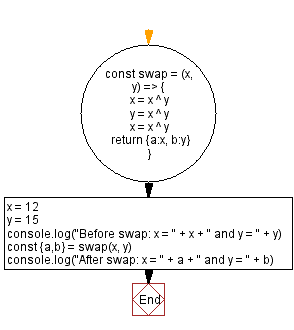# JavaScript - Swap two variables using bit manipulation

## JavaScript Bit Manipulation: Exercise-2 with Solution

Write a JavaScript program to swap two variables using bit manipulation.

Test Data:
(12, 15) -> (15,12)

Sample Solution:

JavaScript Code:

``````const swap = (x, y) => {
x = x ^ y
y = x ^ y
x = x ^ y
return {a:x, b:y}
}
x = 12
y = 15
console.log("Before swap: x = " + x + " and y = " + y)
const {a,b} = swap(x, y)
console.log("After swap: x = " + a + " and y = " + b)
```
```

Sample Output:

```Before swap: x = 12 and y = 15
After swap: x = 15 and y = 12
```

Flowchart:Live Demo:

See the Pen javascript-bit-manipulation-exercise-1 by w3resource (@w3resource) on CodePen.

* To run the code mouse over on Result panel and click on 'RERUN' button.*

Improve this sample solution and post your code through Disqus

What is the difficulty level of this exercise?

Test your Programming skills with w3resource's quiz.

﻿